# Spectral density

(diff) ← Older revision | Latest revision (diff) | Newer revision → (diff)

of a stationary stochastic process or of a homogeneous random field in-dimensional space

The Fourier transform of the covariance function of a stochastic process which is stationary in the wide sense (cf. Stationary stochastic process; Random field, homogeneous). Stationary stochastic processes and homogeneous random fields for which the Fourier transform of the covariance function exists are called processes with a spectral density.

Letbe an-dimensional stationary stochastic process, and letbe its spectral representation (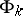is the spectral measure corresponding to the-th component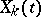of the multi-dimensional stochastic process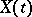). The range of integration is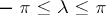in the case of discrete time, and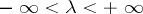in the case of continuous time. The processhas a spectral densityif all the elements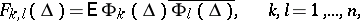of the spectral measure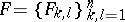are absolutely continuous and if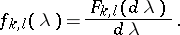In particular, if the relation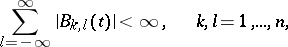holds for,where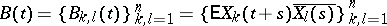is the covariance function of, thenhas a spectral density and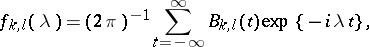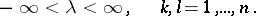The situation is similar in the case of processesin continuous time. The spectral density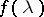is sometimes called the second-order spectral density, in contrast to higher spectral densities (see Spectral semi-invariant).

A homogeneous-dimensional random field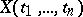has a spectral density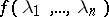if its spectral resolution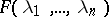possesses the property that its mixed derivativeexists almost-everywhere, and then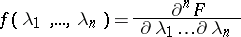and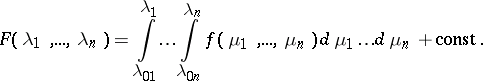How to Cite This Entry:
Spectral density. Encyclopedia of Mathematics. URL: http://encyclopediaofmath.org/index.php?title=Spectral_density&oldid=13166
This article was adapted from an original article by I.G. Zhurbenko (originator), which appeared in Encyclopedia of Mathematics - ISBN 1402006098. See original article